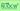# Econometrics ILecturer Tadashi SONODA, Professor School of Economics / Graduate School of Economics, 2010 Fall Economics students (2・1.5 hours / session One session / week 15 weeks / semester)

### Key Features

To increase students' understanding, I try to keep calculations and proof in statistics to a minimum and explain the concepts and technical terms in econometrics using economic examples and illustrations. Due to a lack of Japanese textbooks containing a good balance of appropriate examples and statistical calculations, I instead prepare a handout based on a popular textbook of introductory econometrics which is used in other countries. The handout has some blank spaces left for figures, calculations and proofs which students fill in themselves to increase their understanding.

### Course Aims

We focus on "regression analysis," in which a variable of interest (for example, hourly wage of workers) is explained by other variables (for example, years of schooling, work experience, age, sex), and study a fundamental method called "ordinary least squares". We also study statistical inferences based on this method, in which we ask, for example, "Does the hourly wage of workers increase with their years of schooling?" or "Does the hourly wage differ between male and female workers with the same years of schooling?".

None.

### Course Requirements

It is preferable for students to already know a basic statistical method.

Session Contents
1 What is econometrics? / The nature of economic data
2 Review of basic statistics (1)
3 Simple regression model (1)
4 Simple regression model (2)
5 Simple regression model (3)
6 Multiple regression model: estimation (1)
7 Multiple regression model: estimation (2)
8 Review of basic statistics (2)
9 Multiple regression model: statistical inference (1)
10 Multiple regression model: statistical inference (2)
11 Other fundamental tools in econometrics (1)
12 Other fundamental tools in econometrics (2)
13 Dummy variables
14 Course Review
15 Summary / Final exam